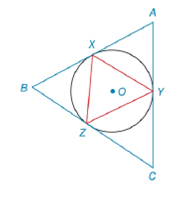Chapter 6.4, Problem 11EElementary Geometry For College St...

7th Edition
Alexander + 2 others
ISBN: 9781337614085

Solutions

Chapter
SectionElementary Geometry For College St...

7th Edition
Alexander + 2 others
ISBN: 9781337614085
Textbook Problem

X , Y , and Z are on circle O such that m X Y ⌢ = 120 ° , m Y Z ⌢ = 130 ° , and m X Z ⌢ = 110 ° . Suppose that triangle X Y Z is drawn and that the triangle A B C is constructed with its sides tangent to circle O at X , Y , and Z . Are △ X Y Z and △ A B C similar triangles?To determine

To find:

If the given two triangles are similar or not.

Explanation

Given:

A circle O and three points X, Y, and Z on the circle such that mXY=120°, mYZ=130°, and mXZ=110°. Also triangle XYZ is drawn and that the triangle ABC is constructed with its sides tangent to circle O at X, Y, and Z. The figure given below

Postulate:

The measure of an inscribed angle of a circle is half the measure of its intercepted arc. Consider the figure below, let the inscribed angle be 2.

Then m2=12mHJ and generally HKKJ.

The measure of the angle formed by intersecting tangents is given by half the positive difference of the measures of the intercepted arcs.

Consider the figure below, let the inscribed angle be 5.

Then m5=12(mRVTmRT) and SR=ST.

Calculation:

Since, the measure of an inscribed angle of a circle is half the measure of its intercepted arc therefore from the figure given,

We get, mXZY=12mXY.

Substituting mXY=120° in mXZY=12mXY,

mXZY=12mXY=12(120°)=60°

Similarly, mZXY=12mYZ.

Substituting mYZ=130° in mZXY=12mYZ,

mZXY=12mYZ=12(130°)=65°

Similarly, mXYZ=12mXZ.

Substituting mXZ=110° in mXYZ=12mXZ,

mXYZ=12mXZ=12(110°)=55°

Since, the measure of the angle formed by intersecting tangents is given by half the positive difference of the measures of the intercepted arcs therefore from the figure given, we get

mABC=12(mXYZmXZ)

The arc XYZ can be expressed as sum of arcs XY and YZ.

Substituting mXYZ=mXY+mYZ in mABC=12(mXYZmXZ),

mABC=12[(mXY+mYZ)mXZ]

Substituting mXY=120°, mXZ=110°, mYZ=130° in above equation,

mABC=12[(120°+130°)110°]

Simplifying,

mABC=12(250°110°)=12(140)=70°

Similarly, mBAC=12(mXZYmXY)

Still sussing out bartleby?

Check out a sample textbook solution.

See a sample solution

The Solution to Your Study Problems

Bartleby provides explanations to thousands of textbook problems written by our experts, many with advanced degrees!

Get Started